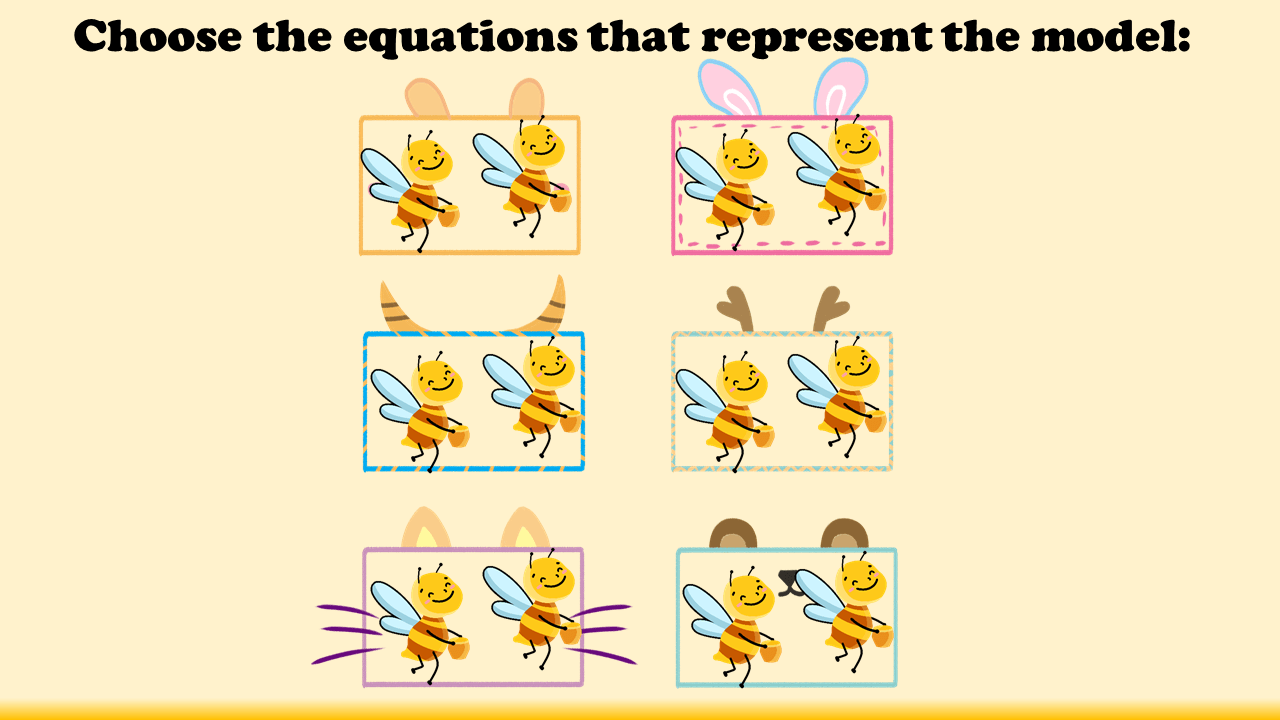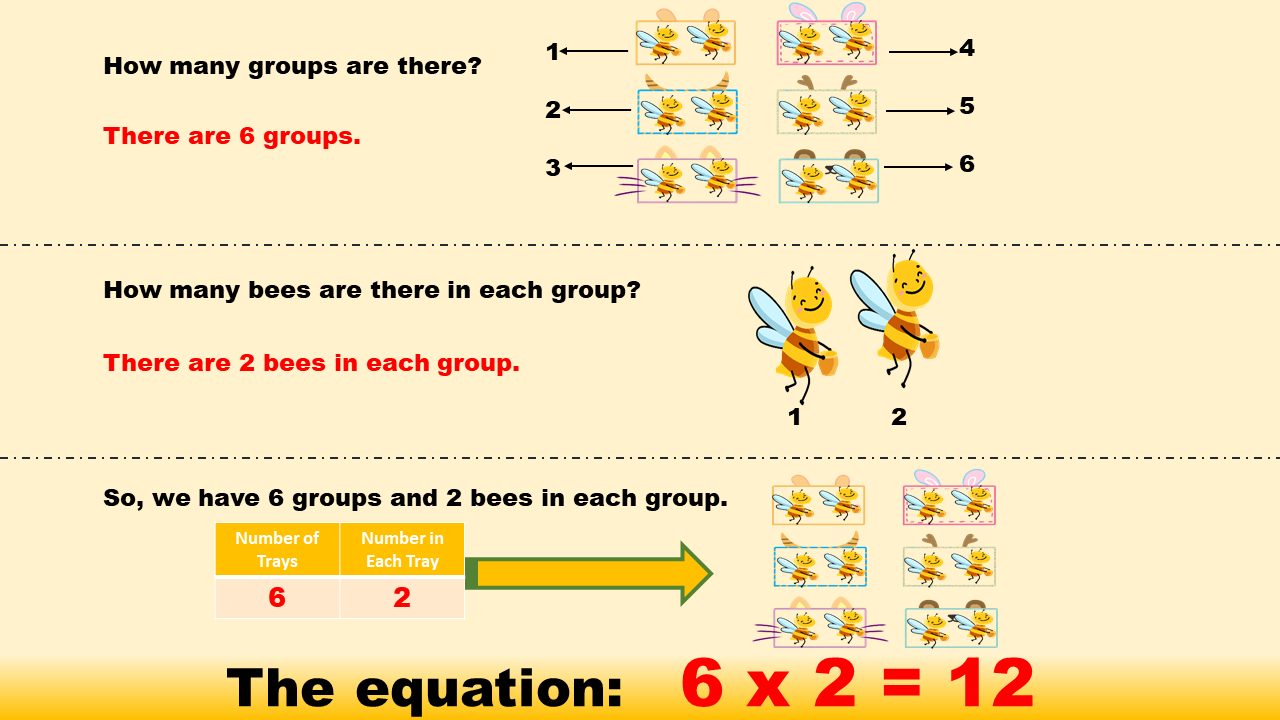1
visibility
9 - Easy(Multiplication)• A
9 - Easy(Multiplication)

6 × 2 = 12

• B
9 - Easy(Multiplication)

6 × 1 = 6

• C
9 - Easy(Multiplication)

2 × 5 = 10

• D
9 - Easy(Multiplication)

5 × 1 = 5